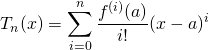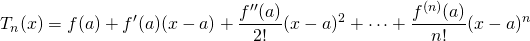# Taylor Polynomial: Formula & Examples

Lesson Transcript
Instructor
Shaun Ault

Shaun is currently an Assistant Professor of Mathematics at Valdosta State University as well as an independent private tutor.

Expert Contributor
Elaine Chan

Dr. Chan has a Ph.D. from the U. of California, Berkeley. She has done research and teaching in mathematics and physical sciences.

In this lesson we explore the Taylor polynomial, which serves as a way to approximate a given function near a given point. Two examples will be worked out in detail. Updated: 08/11/2020

## What Is a Taylor Polynomial?

Let's start with the definition. Given a function f, a specific point x = a (called the center), and a positive integer n, the Taylor polynomial of f at a, of degree n, is the polynomial T of degree n that best fits the curve y = f(x) near the point a, in the sense that T and all its first n derivatives have the same value at x = a as f does.

If the center is 0, then T may be called a Maclaurin polynomial.

Appearing here is the formula to find the Taylor polynomial:Equivalently, if you expand the sum out, you see something like this one appearing here:Now we know it may look daunting at first, but there's a step-by-step procedure for creating a Taylor polynomial. As long as you've had plenty of experience with derivatives, and if you know your way around factorials (that's the i! in the formula), then it shouldn't be too hard.

An error occurred trying to load this video.

Try refreshing the page, or contact customer support.

Coming up next: Taylor Series: Definition, Formula & Examples

### You're on a roll. Keep up the good work!

Replay
Your next lesson will play in 10 seconds
• 0:04 What Is a Taylor Polynomial?
• 1:03 Computing a Taylor Polynomial
• 2:44 Another Example
• 3:36 Taylor Polynomials: Functions
• 4:38 Lesson Summary
Save Save

Want to watch this again later?

Log in or sign up to add this lesson to a Custom Course.

Timeline
Autoplay
Autoplay
Speed Speed Audio mode

## Computing a Taylor Polynomial

Notice that each term of the formula requires:

1. A derivative of some order. In fact, the little (i) notation on the f means the derivative of order i (it doesn't mean f to the i power, even though the little (i) seems to look like an exponent).
2. Plugging the given value a into that derivative.
3. Dividing by the factorial number i!. Recall that i! = i(i - 1)(i - 2). . . (2)(1), if i > 0, and, by convention, 0! = 1.
4. Multiplying by (x - a) to the i power. Note, in this formula x is just an unknown variable, and we leave it in the expression as part of the polynomial.

Once each term is built up, then the entire Taylor polynomial may be read off as the sum of those terms. Let's see this by example!

Example: Find the third degree Taylor polynomial for f(x) = 4/x, centered at x = 1.

First, we rewrite 4/x = 4x(-1) to make derivatives easier to find. Notice the table appearing on your screen right now starts with i = 0.

i i-th deriv. Plug in center (a) Divide by i! Mult. by (x - a)i
0 4x(-1) 4(1)(-1) = 4 4/0! = 4/1 = 4 4
1 -4x(-2) -4(1)(-2) = -4 -4/1! = -4/1 = -4 -4(x - 1)
2 8x(-3) 8(1)(-3) = 8 8/2! = 8/2 = 4 4(x - 1)2
3 -24x(-4) -24(1)(-4) = -24 -24/3! = -24/6 = -4 -4(x - 1)3

Therefore, you should always start with 0. In fact, it's the easiest part of the table, because this term simply boils down to f(a). Here, a = 1, because that's the given center.

So the answer is: T = 4 - 4(x - 1) + 4(x - 1)2 - 4(x - 1)3.

## Another Example

Probably one of the most important functions in calculus is the natural exponent, ex, or as it is often written, exp(x). What makes this function so special is that the only function whose derivative is equal to itself is a constant multiple of exp(x). This fact makes finding Taylor polynomials of exp(x) quite easy!

So here's our example: Find the fifth degree Maclaurin polynomial for exp(x).

As you look over this table, recall that a Maclaurin polynomial is simply a Taylor polynomial centered at a = 0.

To unlock this lesson you must be a Study.com Member.
Create your account

Additional Activities

## Function Represented by the Sum of a Taylor Series

Tn (x) is the nth degree Taylor polynomial expanded around x = a

If f(x) = Tn (x) + Rn (x) and, in the limit, as n approaches infinity, Rn (x) approaches zero on the interval |x - a| < D, then f(x) can be represented by the sum of the Taylor series on the interval |x-a| < D.

Taylor's Inequality for the remainder:

- M |x-a|n + 1 / (n+1)! ≤ Rn (x) ≤ M |x-a|n + 1 / (n+1)!

Where |f(n + 1) (x)| ≤ M on the interval |x-a| < D.

#### Question :

Find the Maclaurin series for sin(x) and prove that it represents the function for all x.

#### Answer:

Computing the Maclaurin series:

f(x)=sin(x) ; f(0) = 0

f'(x) = cos(x) ; f'(0) = 1

f(x) = -sin(x) ; f(0) = 0

f'(x) = -cos(x) ; f'(0) = -1

f""(x) = sin(x) ; f""(0) = 0

The series is:

f(0) + f'(0)/1! + f(0)/2! + f'(0)/3! + ... =

= x - x3 /3! + x5 /5! + ... =

=Σ (-1)n x2n + 1 / (2n + 1)!

Since the absolute value of all derivatives in the Taylor inequality for the remainder is less than one, we can use M =1.

- |x|n + 1 / (n+1)! ≤ Rn (x) ≤ |x|n + 1 / (n+1)!

In the limit, as n becomes infinite, the remainder approaches zero.

The function can be represented by its series expansion for all values of x.

### Register to view this lesson

Are you a student or a teacher?

### Unlock Your Education

#### See for yourself why 30 million people use Study.com

##### Become a Study.com member and start learning now.
Back
What teachers are saying about Study.com
Create an account to start this course today
Used by over 30 million students worldwide